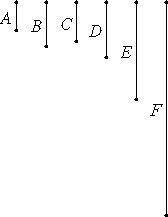# Proposition 27

If two numbers are relatively prime, and each multiplied by itself makes a certain number, then the products are relatively prime; and, if the original numbers multiplied by the products make certain numbers, then the latter are also relatively prime.

Let A and B be two relatively prime numbers, let A multiplied by itself make C, and multiplied by C make D, and let B multiplied by itself make E, and multiplied by E make F.I say that C and E are relatively prime, and that D and F are relatively prime.

VII.25

Since A and B are relatively prime, and A multiplied by itself makes C, therefore C and B are relatively prime.

Since, then, C and B are relatively prime, and B multiplied by itself makes E, therefore C and E are relatively prime.

Again, since A and B are relatively prime, and B multiplied by itself makes E, therefore A and E are relatively prime.

VII.26

Since, then, the two numbers A and C are relatively prime to the two numbers B and E, both to each, therefore the product of A and C is relatively prime to the product of B and E. And the product of A and C is D, and the product of B and E is F.

Therefore D and F are relatively prime.

Therefore, if two numbers are relatively prime, and each multiplied by itself makes a certain number, then the products are relatively prime; and, if the original numbers multiplied by the products make certain numbers, then the latter are also relatively prime.

Q.E.D.

## Guide

The proposition states that

if a and b are relatively prime, then an and bn are relatively prime.

Euclid’s proof only covers the cases where n is 2 or 3, but the way it is used in VIII.2, any powers need to be relatively prime.

The proof of this proposition uses the last two propositions. Assume that a and b are relatively prime. Then applying VII.25 twice, we first get a2 and b relatively prime, then we get a2 and b2 relatively prime.

Again, by VII.25, a and b2 are relatively prime. Now, a is relatively prime to b2, and b is relatively prime to a2, so by VII.26, a3 is relatively prime to b3.

Likewise, higher powers of a and b can be shown to be relatively prime.

#### Use of this proposition

This proposition is used in VIII.2 and VIII.3.